Image Processing Toolbox User's Guideimhist

Display histogram of image data

Syntax

• ```imhist(I,n)
imhist(X,map)
[counts,x] = imhist(...)
```

Description

`imhist(I)` displays a histogram for the intensity image `I` above a grayscale colorbar. The number of bins in the histogram is specified by the image type. If `I` is a grayscale image, `imhist` uses a default value of 256 bins. If `I` is a binary image, `imhist` uses 2 bins.

`imhist(I,n)` displays a histogram where `n` specifies the number of bins used in the histogram. `n` also specifies the length of the colorbar. If I is a binary image, `n` can only have the value 2.

`imhist(X,map)` displays a histogram for the indexed image `X`. This histogram shows the distribution of pixel values above a colorbar of the colormap `map`. The colormap must be at least as long as the largest index in `X`. The histogram has one bin for each entry in the colormap.

`[counts,x] = imhist(...)` returns the histogram counts in `counts` and the bin locations in `x` so that `stem(x,counts)` shows the histogram. For indexed images, it returns the histogram counts for each colormap entry; the length of `counts` is the same as the length of the colormap.

 Note    For intensity images, the `n` bins of the histogram are each half-open intervals of width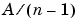. In particular, for intensity images that are not `int16`, the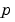th bin is the half-open interval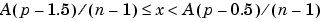, where x is the intensity value. For `int16` intensity images, theth bin is the half-open interval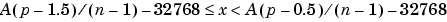, where x is the intensity value. The scale factor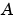depends on the image class.is 1 if the intensity image is `double` or `single`,is 255 if the intensity image is `uint8`, andis 65535 if the intensity image is `uint16` or `int16`.

Class Support

An input intensity image can be of class `uint8`, `uint16`, `int16`, `single`, `double`, or `logical`. An input indexed image can be of class `uint8`, `uint16`, `single`, `double`, or `logical`.

Example

• ```I = imread('pout.tif');
imhist(I)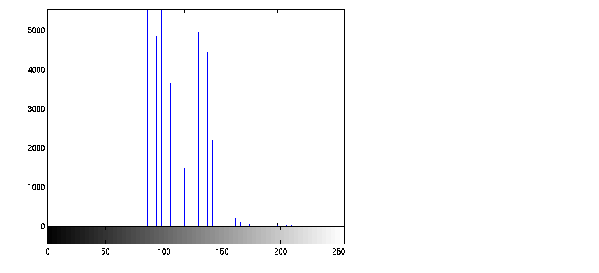```

`histeq`
`hist` in the MATLAB Function Reference